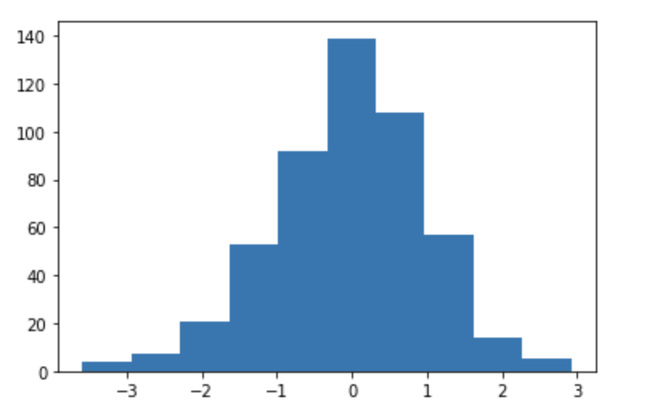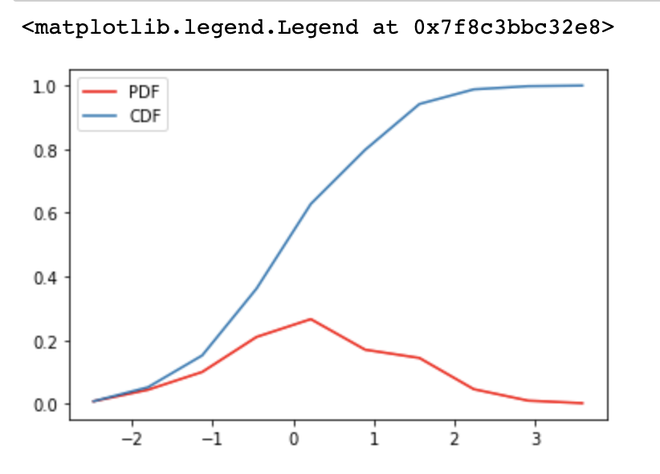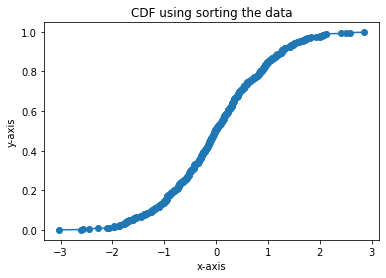Open in App
Not now

# How to calculate and plot a Cumulative Distribution function with Matplotlib in Python ?

• Last Updated : 24 Jan, 2021

Prerequisites: Matplotlib

Matplotlib is a library in Python and it is a numerical — mathematical extension for the NumPy library.  The cumulative distribution function (CDF) of a real-valued random variable X, or just distribution function of X, evaluated at x, is the probability that X will take a value less than or equal to x.

Properties of CDF:

• Every cumulative distribution function F(X) is non-decreasing
• If maximum value of the cdf function is at x, F(x) = 1.
• The CDF ranges from 0 to 1.

### Method 1: Using the histogram

CDF can be calculated using PDF (Probability Distribution Function). Each point of random variable will contribute cumulatively to form CDF.

Example :

A combination set containing 2 balls which can be either red or blue can be in the following set.

{RR, RB, BR, BB}

t -> No of red balls.

P(x = t) -> t = 0 : 1 / 4 [BB]

t = 1 : 2 / 4 [RB, BR]

t = 2 : 1 / 4 [RR]

CDF :

F(x) = P(x<=t)

x = 0 : P(0)               -> 1 / 4

x = 1 : P(1) + P(0)        -> 3 / 4

x = 2 : P(2) + P(1) + P(0) -> 1

Approach

• Import modules
• Declare number of data points
• Initialize random values
• Plot histogram using above data
• Get histogram data
• Finding PDF using histogram data
• Calculate CDF
• Plot CDF

Example:

## Python3

 `# defining the libraries``import` `numpy as np``import` `matplotlib.pyplot as plt``import` `pandas as pd``%``matplotlib inline`` ` `# No of Data points``N ``=` `500`` ` `# initializing random values``data ``=` `np.random.randn(N)`` ` `# getting data of the histogram``count, bins_count ``=` `np.histogram(data, bins``=``10``)`` ` `# finding the PDF of the histogram using count values``pdf ``=` `count ``/` `sum``(count)`` ` `# using numpy np.cumsum to calculate the CDF``# We can also find using the PDF values by looping and adding``cdf ``=` `np.cumsum(pdf)`` ` `# plotting PDF and CDF``plt.plot(bins_count[``1``:], pdf, color``=``"red"``, label``=``"PDF"``)``plt.plot(bins_count[``1``:], cdf, label``=``"CDF"``)``plt.legend()`

Output:

Histogram plot of the PDF and CDF :Plotted CDF:CDF plotting

### Method 2: Data sort

This method depicts how CDF can be calculated and plotted using sorted data. For this, we first sort the data and then handle further calculations.

Approach

• Import module
• Declare number of data points
• Create data
• Sort data in ascending order
• Get CDF
• Plot CDF
• Display plot

Example:

## Python3

 `# defining the libraries``import` `numpy as np``import` `matplotlib.pyplot as plt``import` `pandas as pd``%``matplotlib inline`` ` `# No of data points used``N ``=` `500`` ` `# normal distribution``data ``=` `np.random.randn(N)`` ` `# sort the data in ascending order``x ``=` `np.sort(data)`` ` `# get the cdf values of y``y ``=` `np.arange(N) ``/` `float``(N)`` ` `# plotting``plt.xlabel(``'x-axis'``)``plt.ylabel(``'y-axis'``)`` ` `plt.title(``'CDF using sorting the data'``)`` ` `plt.plot(x, y, marker``=``'o'``)`

Output:My Personal Notes arrow_drop_up